# Punjab Board (PSEB) Question Paper for Class 10th Maths 2018 In PDF

## PSEB Matriculation (10th Standard) Maths Exam Question Papers 2018 with Solutions – Free Download

PSEB (Punjab board) Annual Exam Class 10 maths 2018 Question Paper (C) with solutions are given at BYJU’S with detailed explanations. All the solutions of pseb class 10 Maths previous year question papers are available in a downloadable pdf format as well as in a scrollable image format so that students can access them easily. Practicing more question papers of PSEB Class 10 Maths annual exam papers will help the students in improving their confidence on a successful attempt of the final exam. Mathematics is a subject in which students can score full marks just by memorising all the Maths formulas of Class 10 and theorems. Students can also solve and practice Punjab Board (PSEB) Question Paper for Class 10th Maths 2018 In PDF with solutions to prepare effectively for the exams.

### PSEB Class 10 Mathematics Question Paper 2018 Paper 4C with Solutions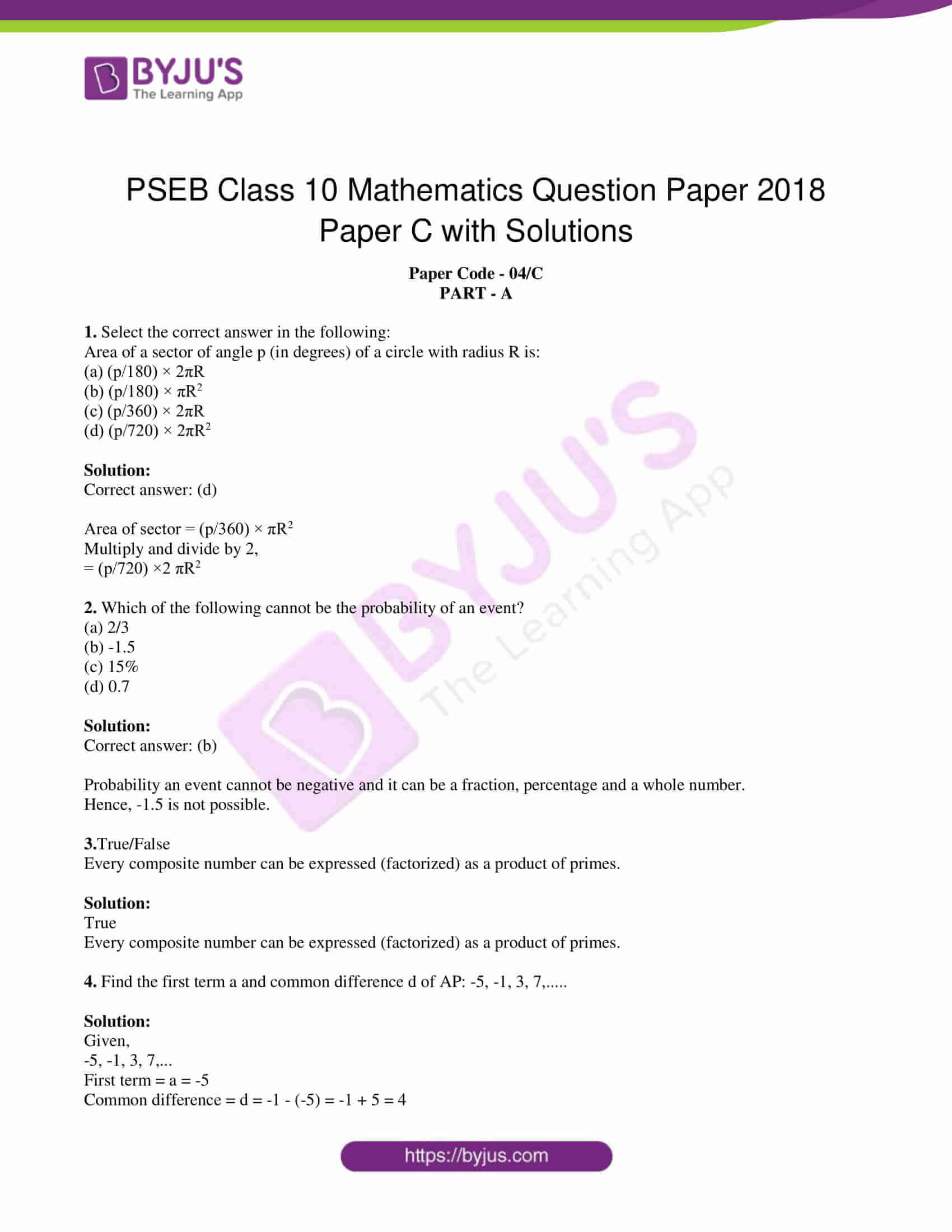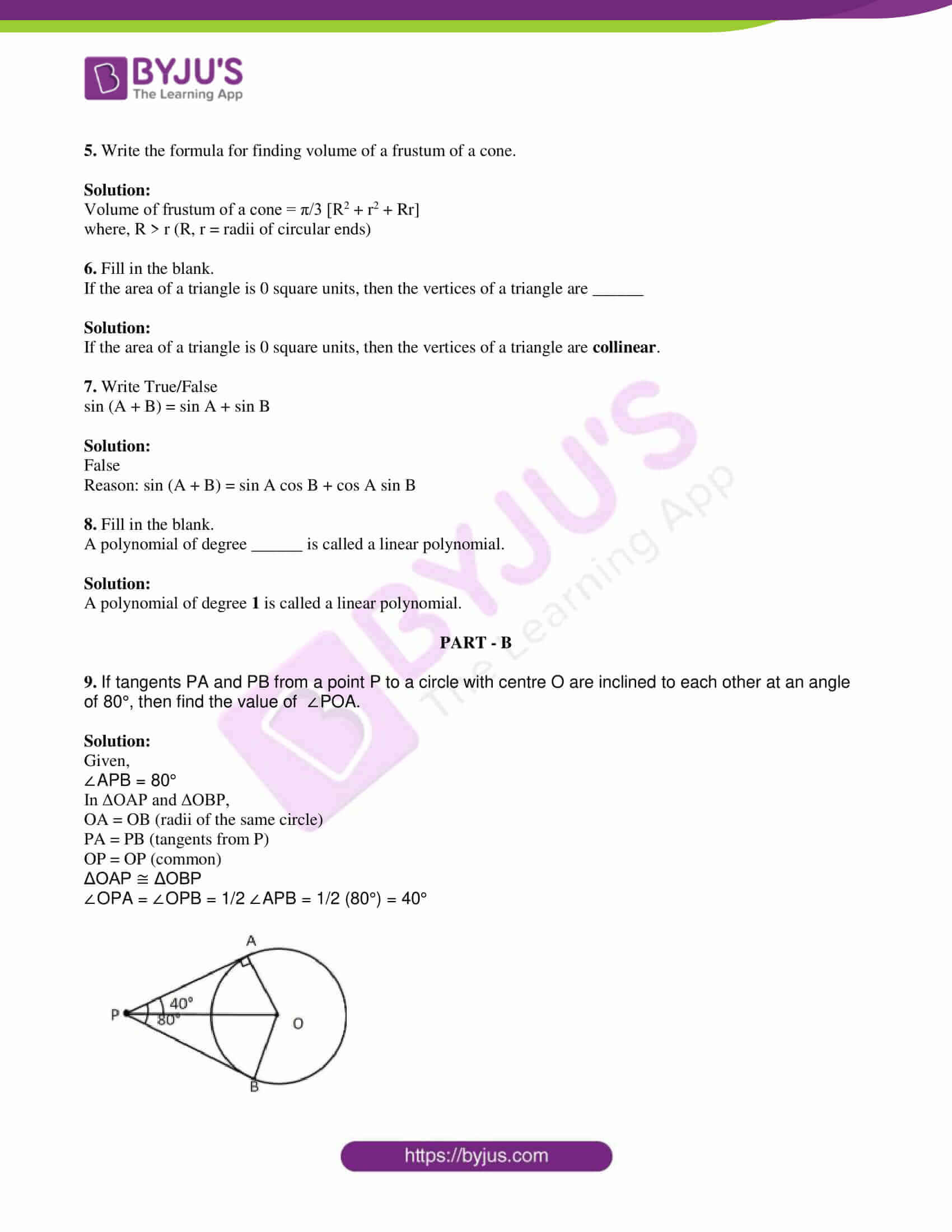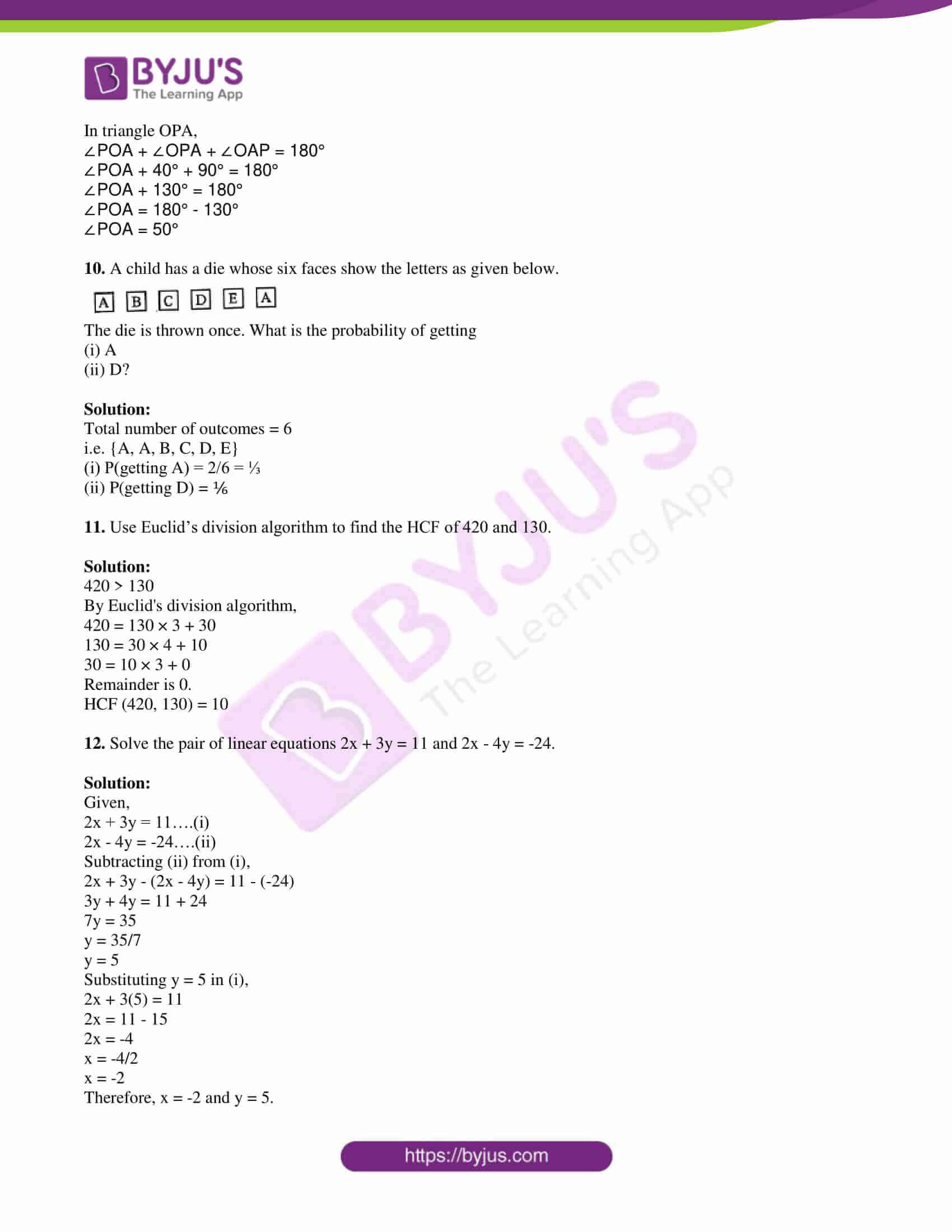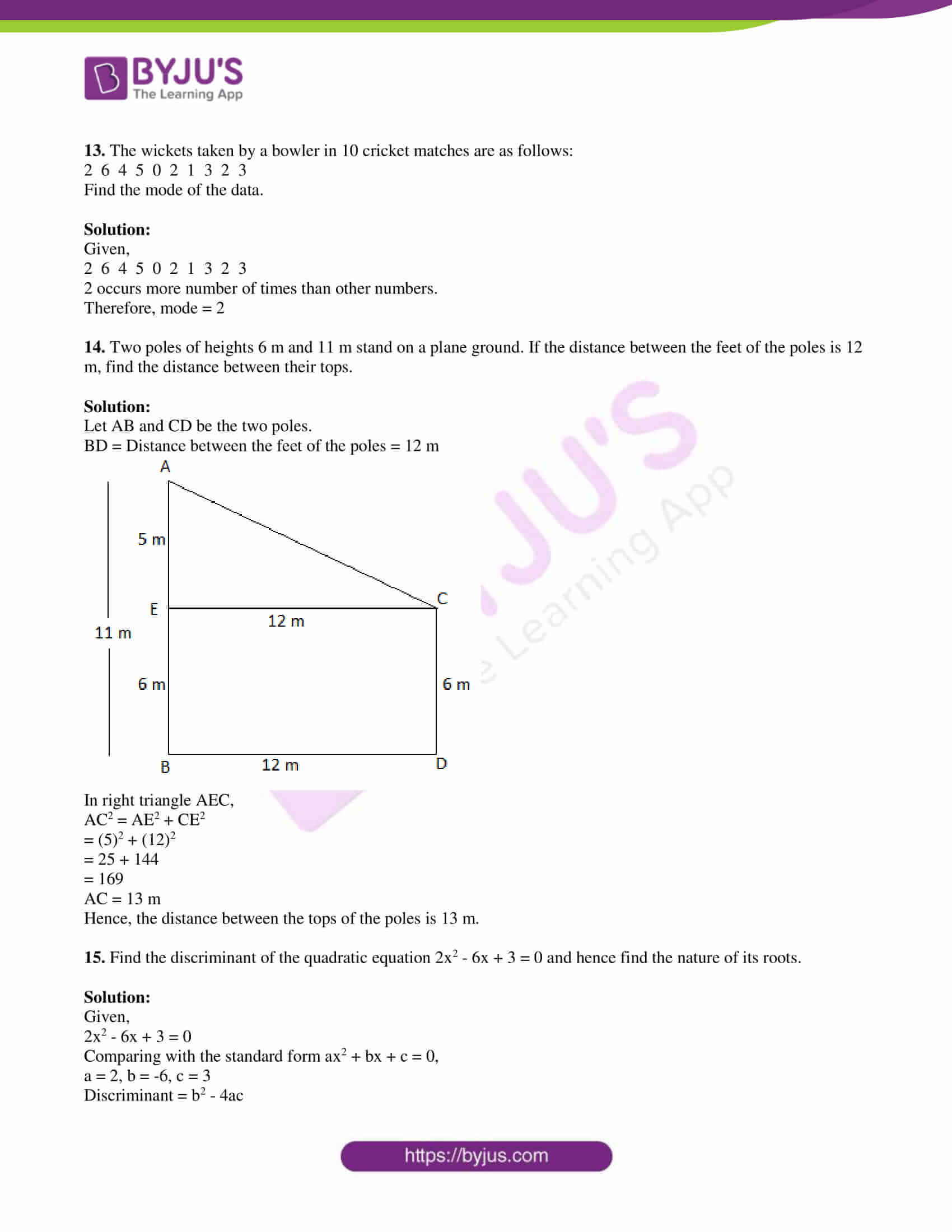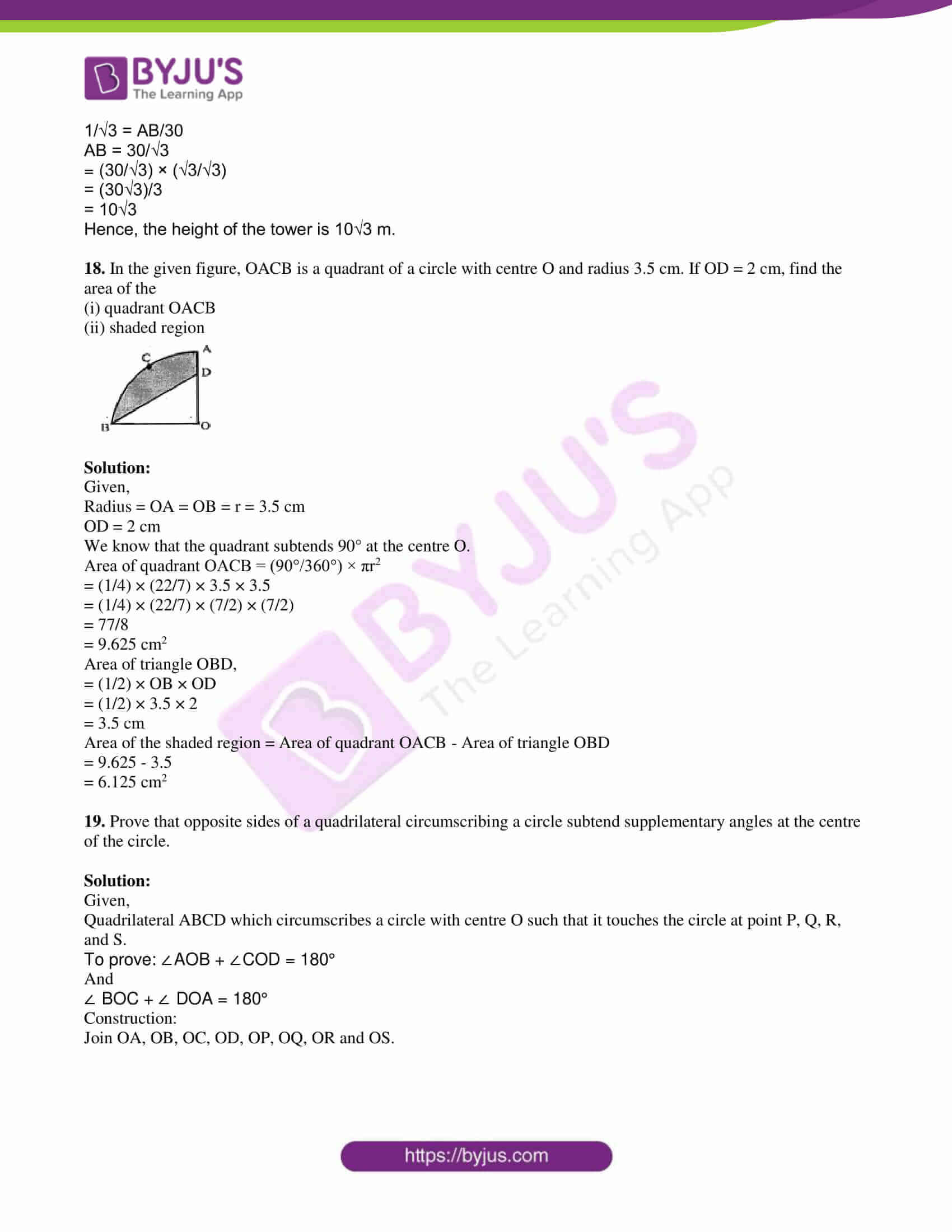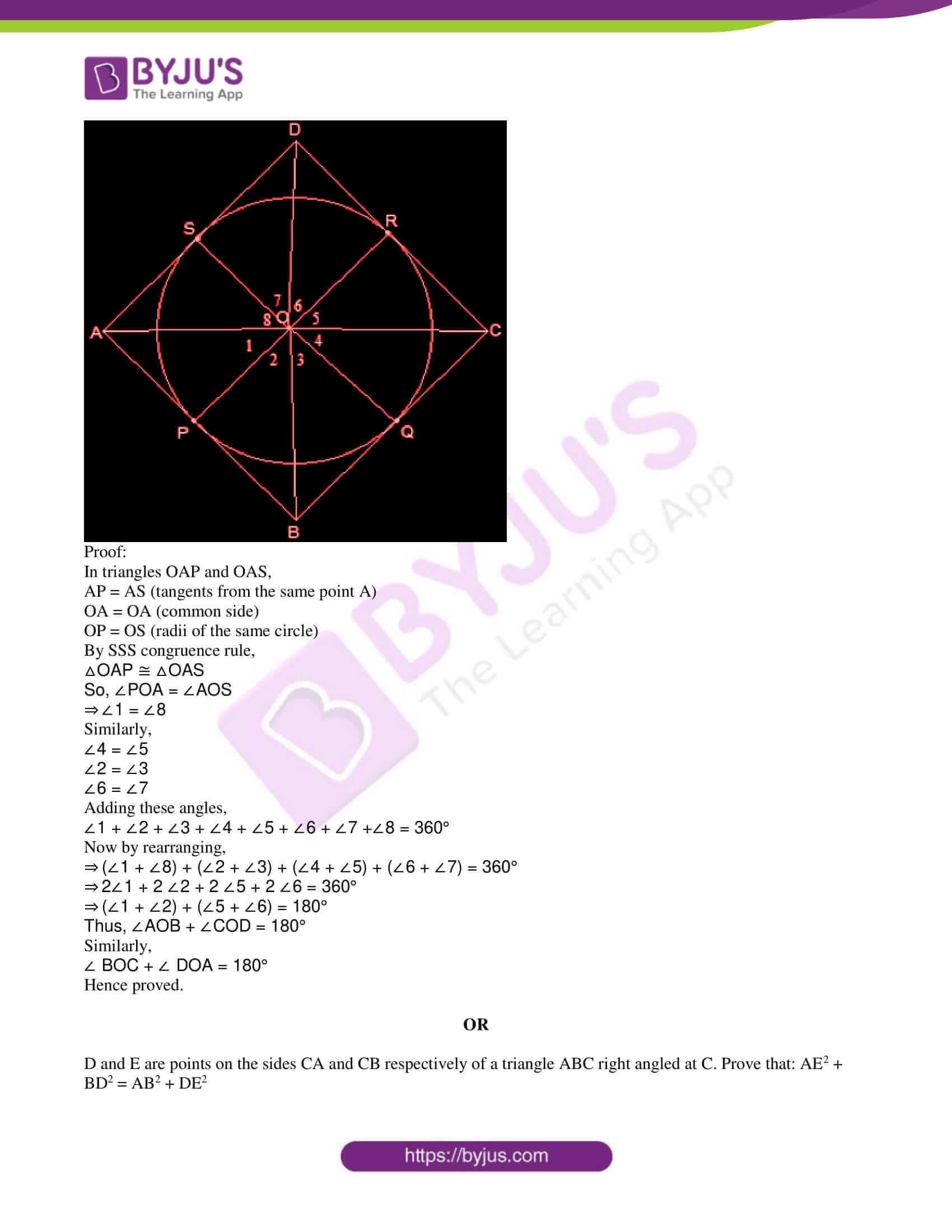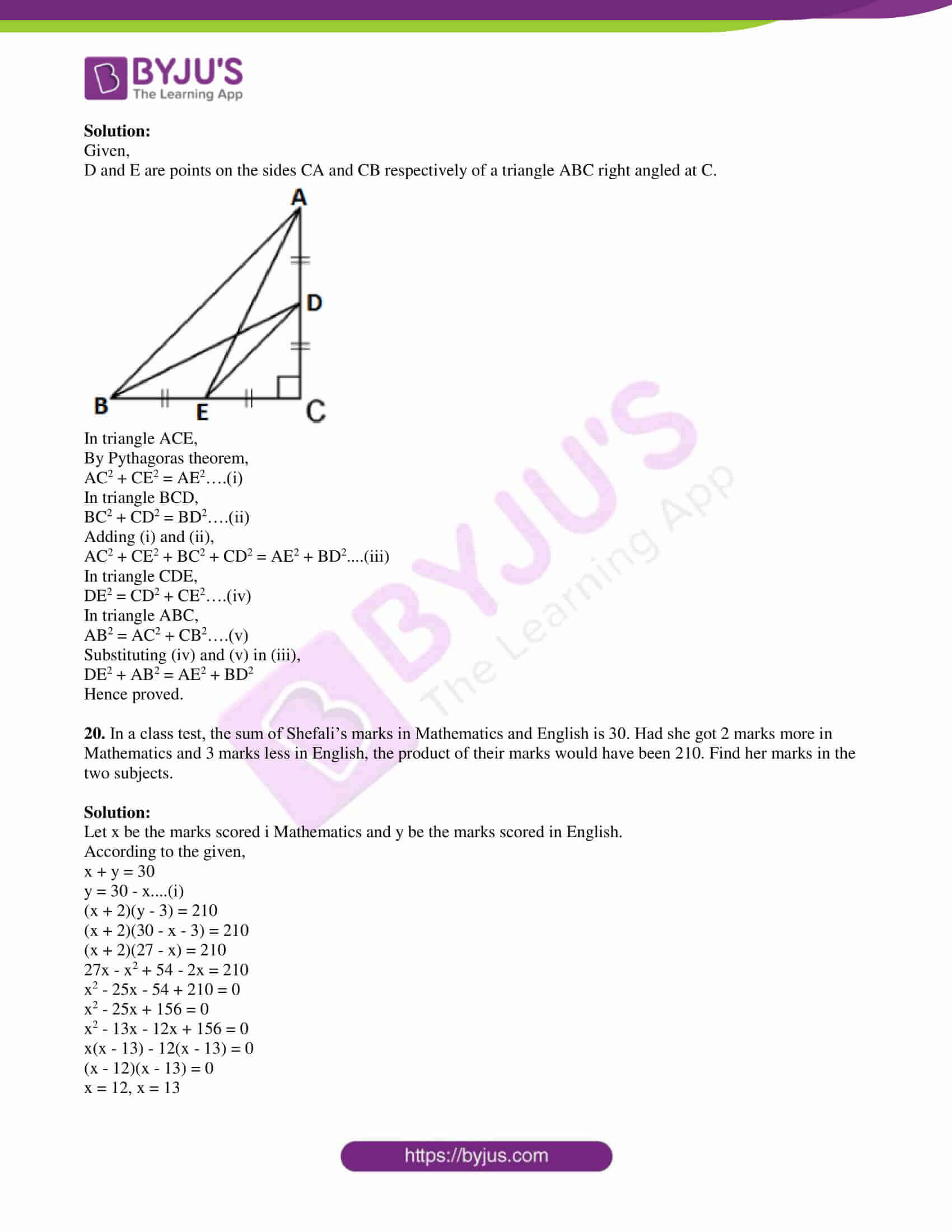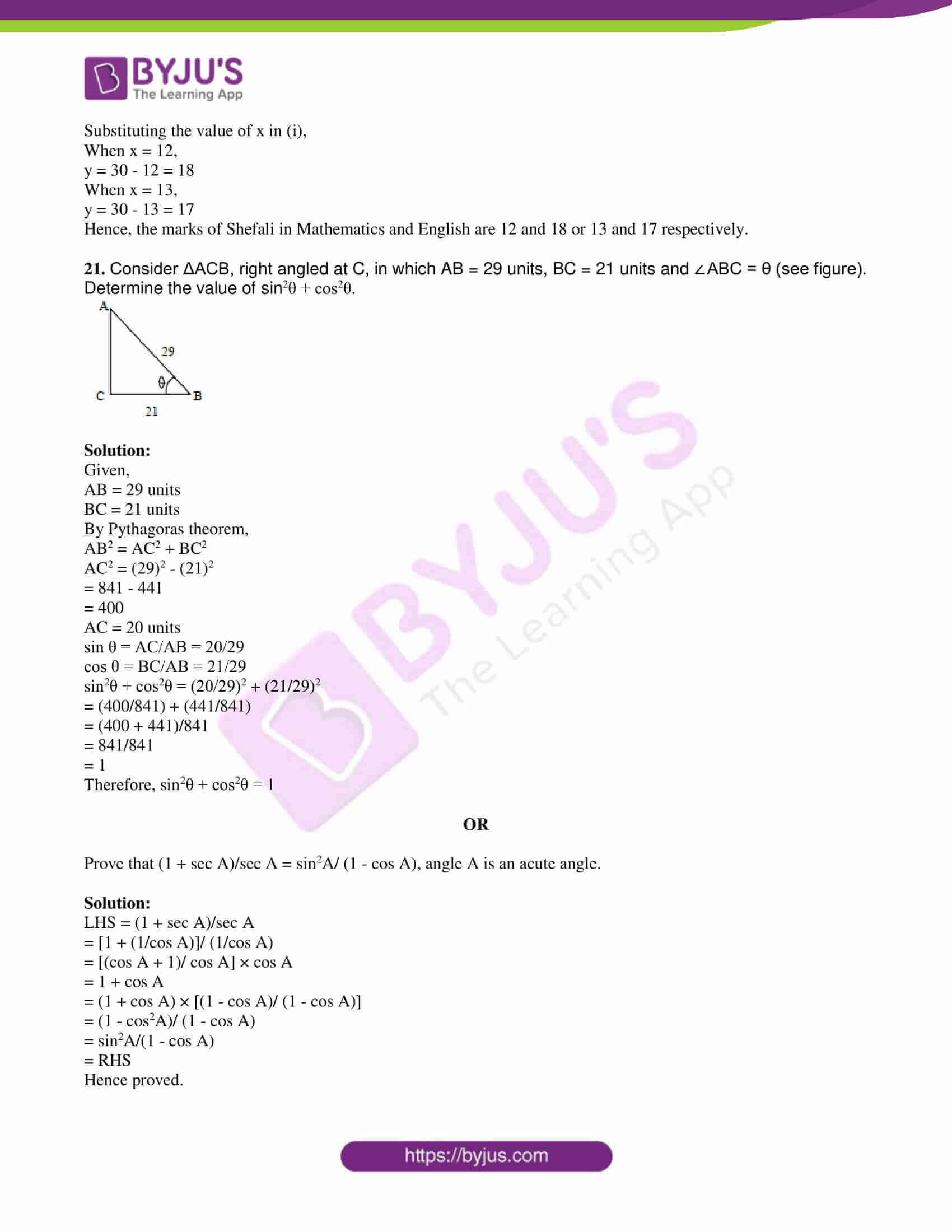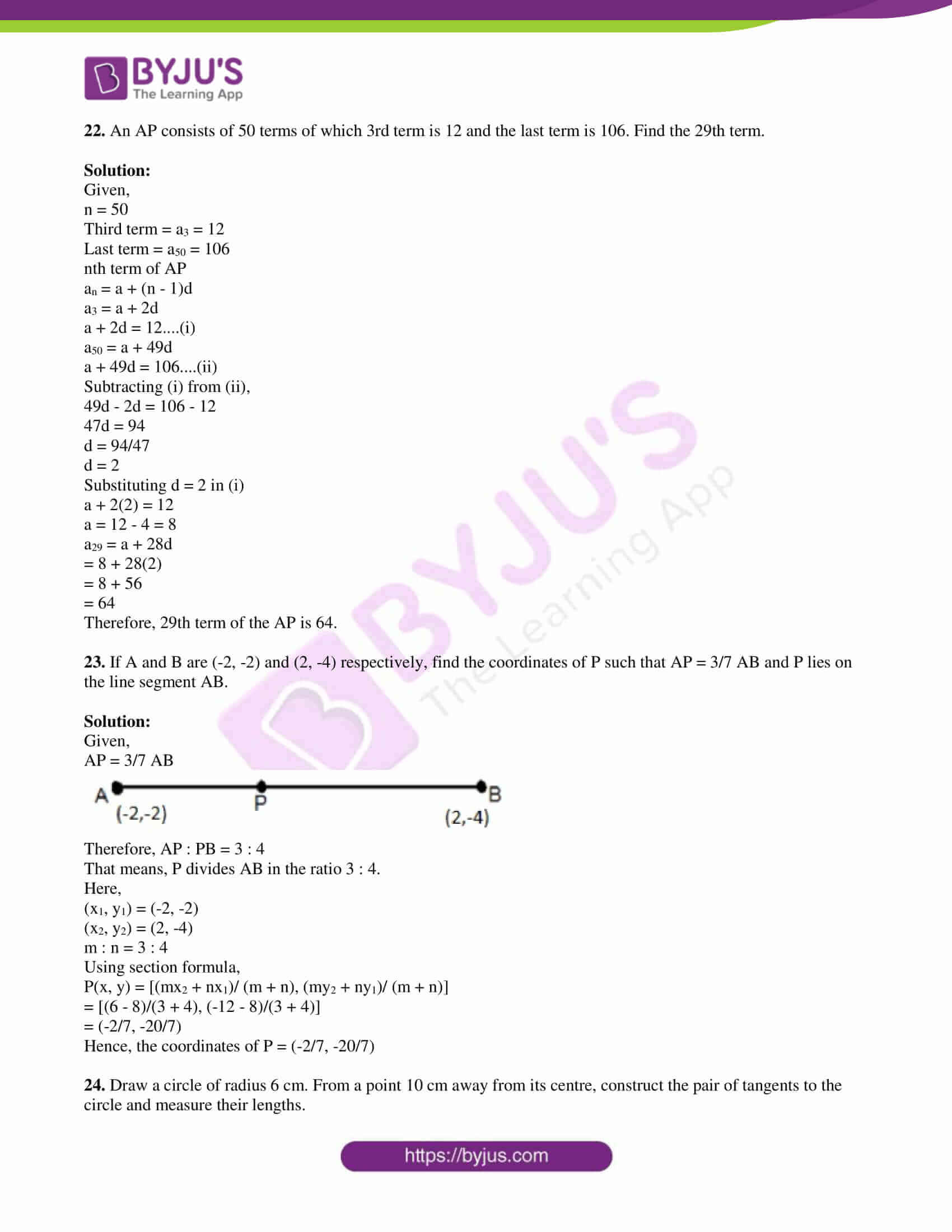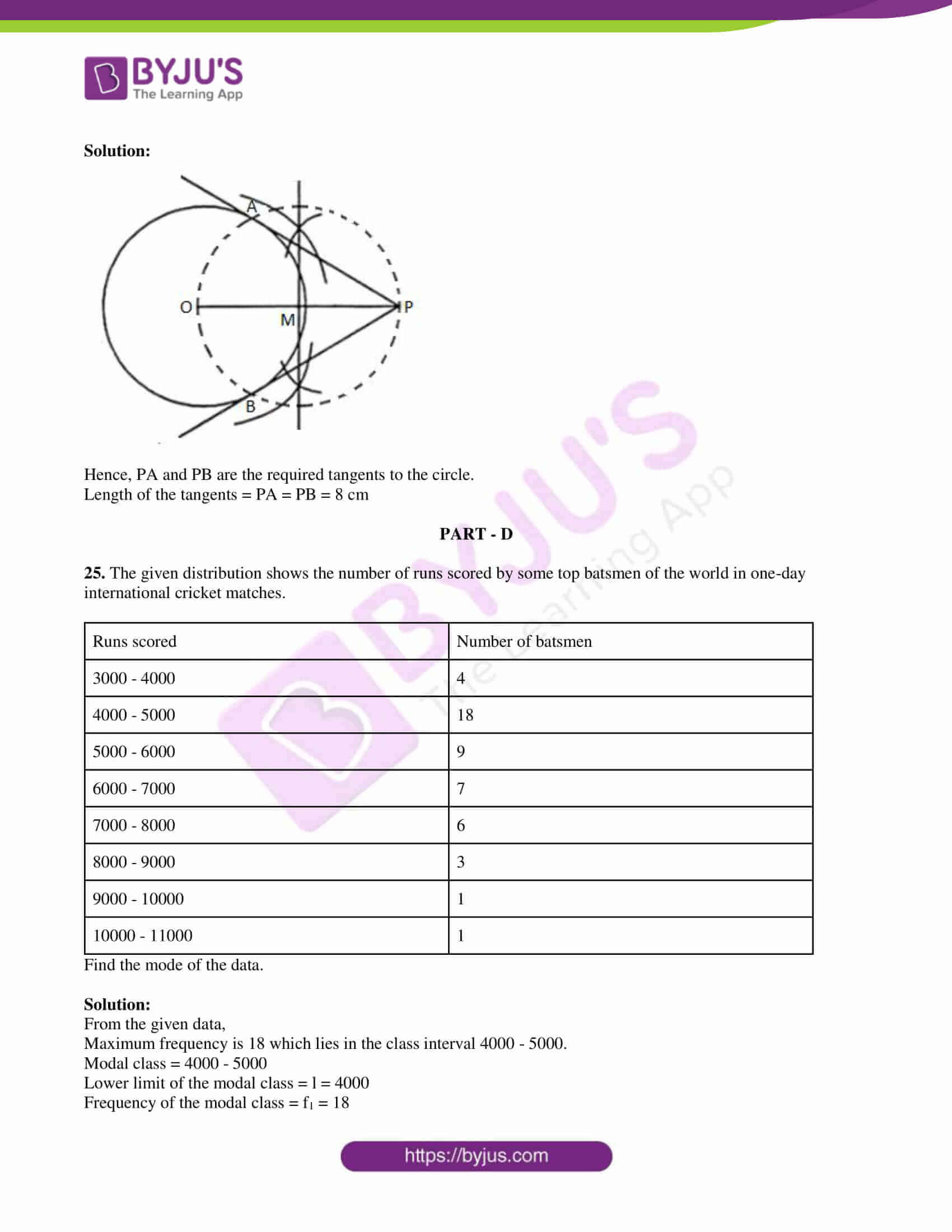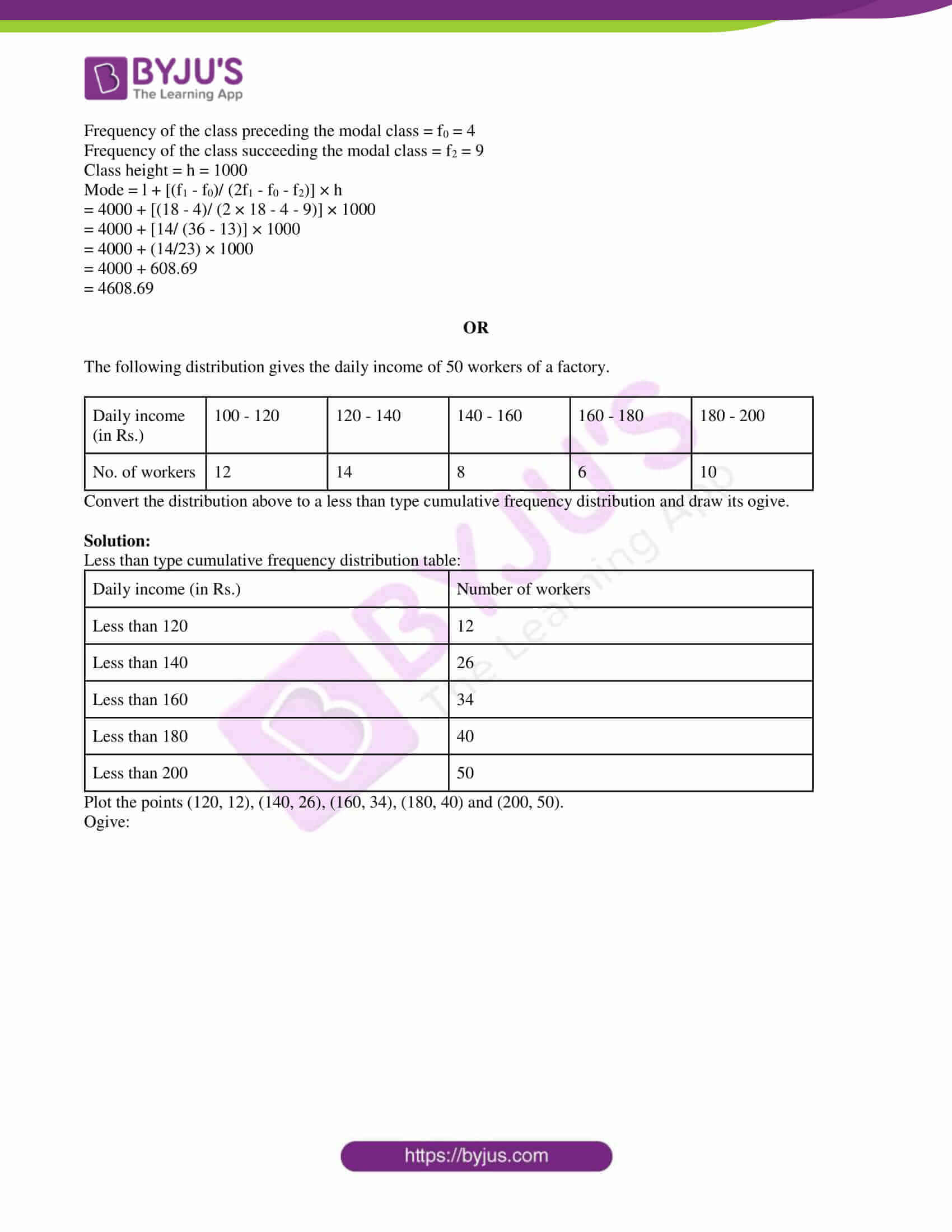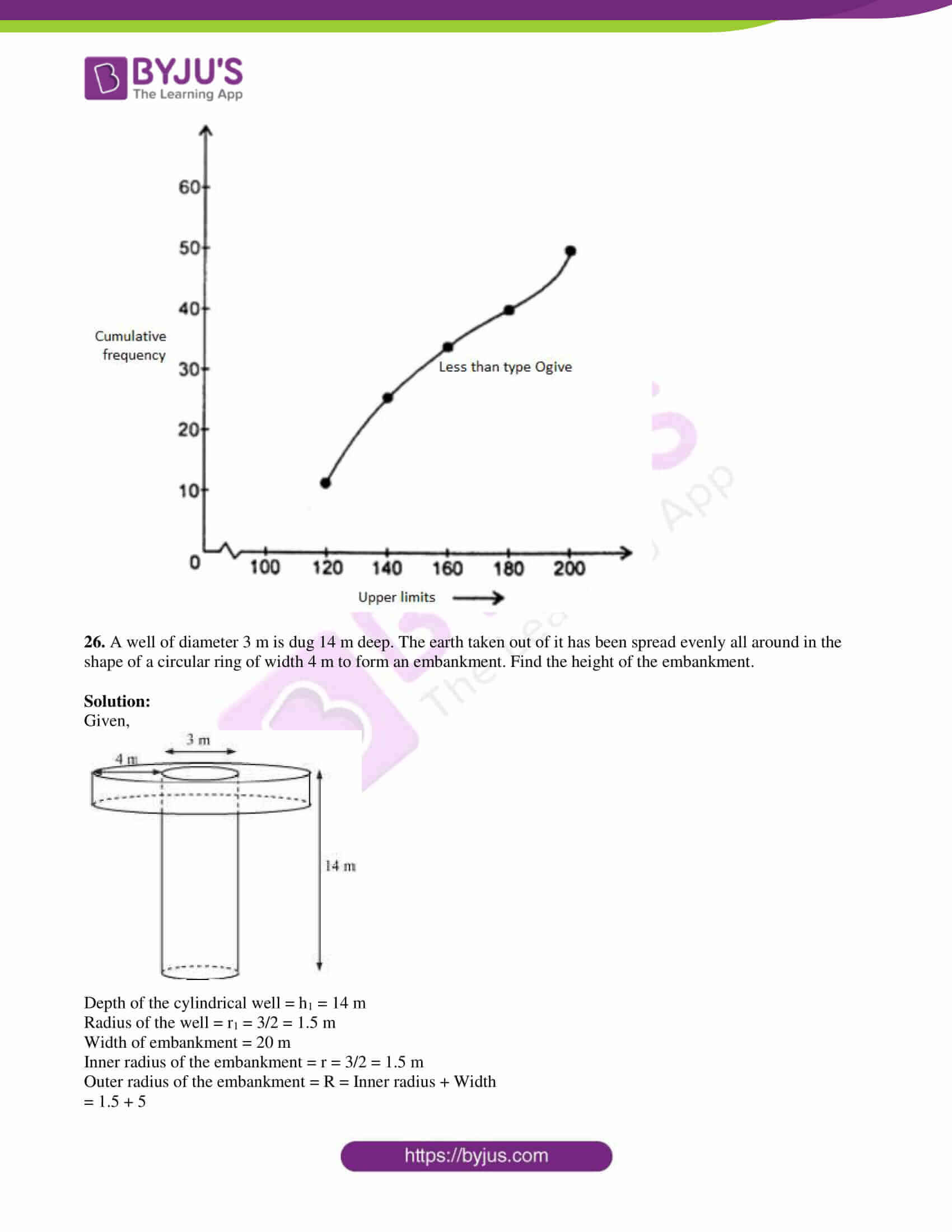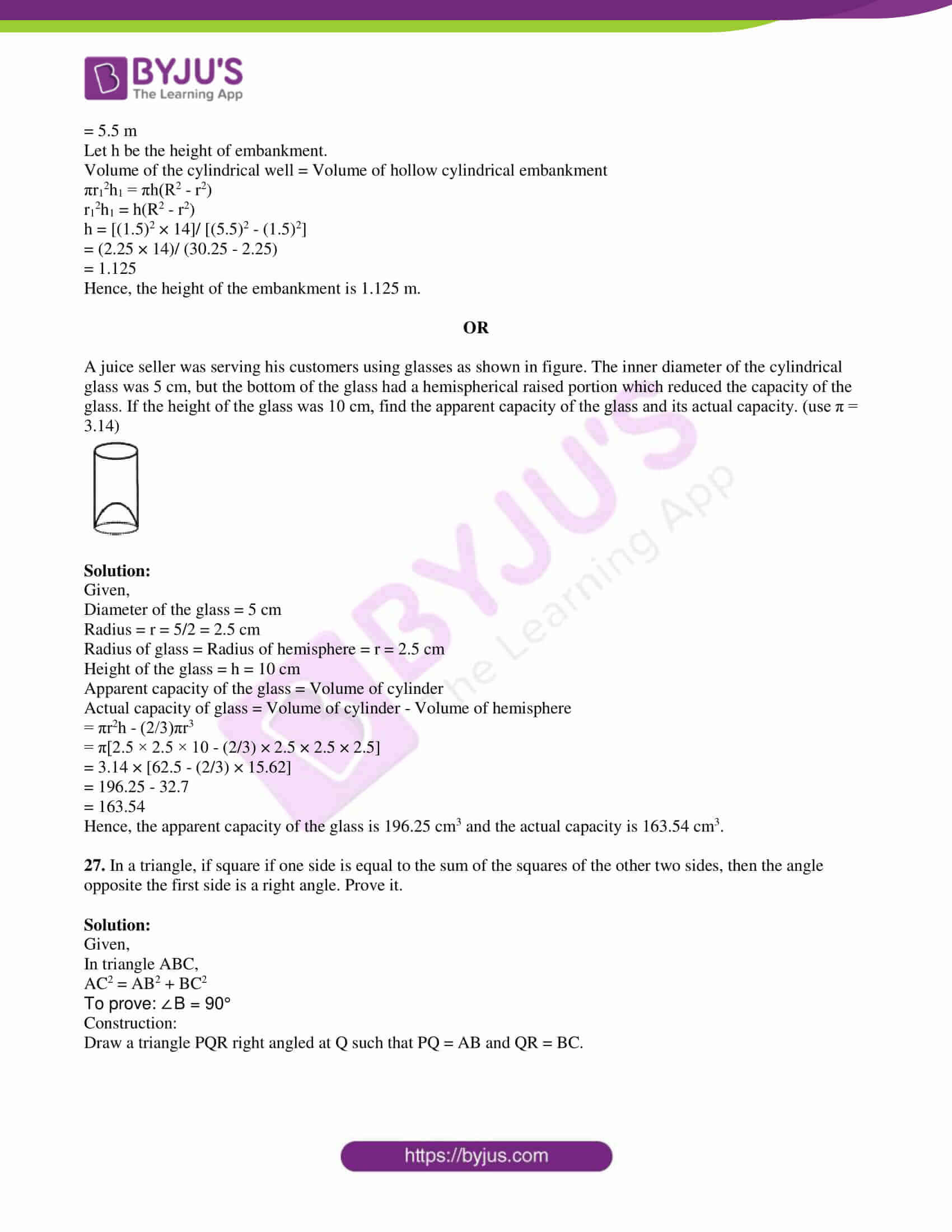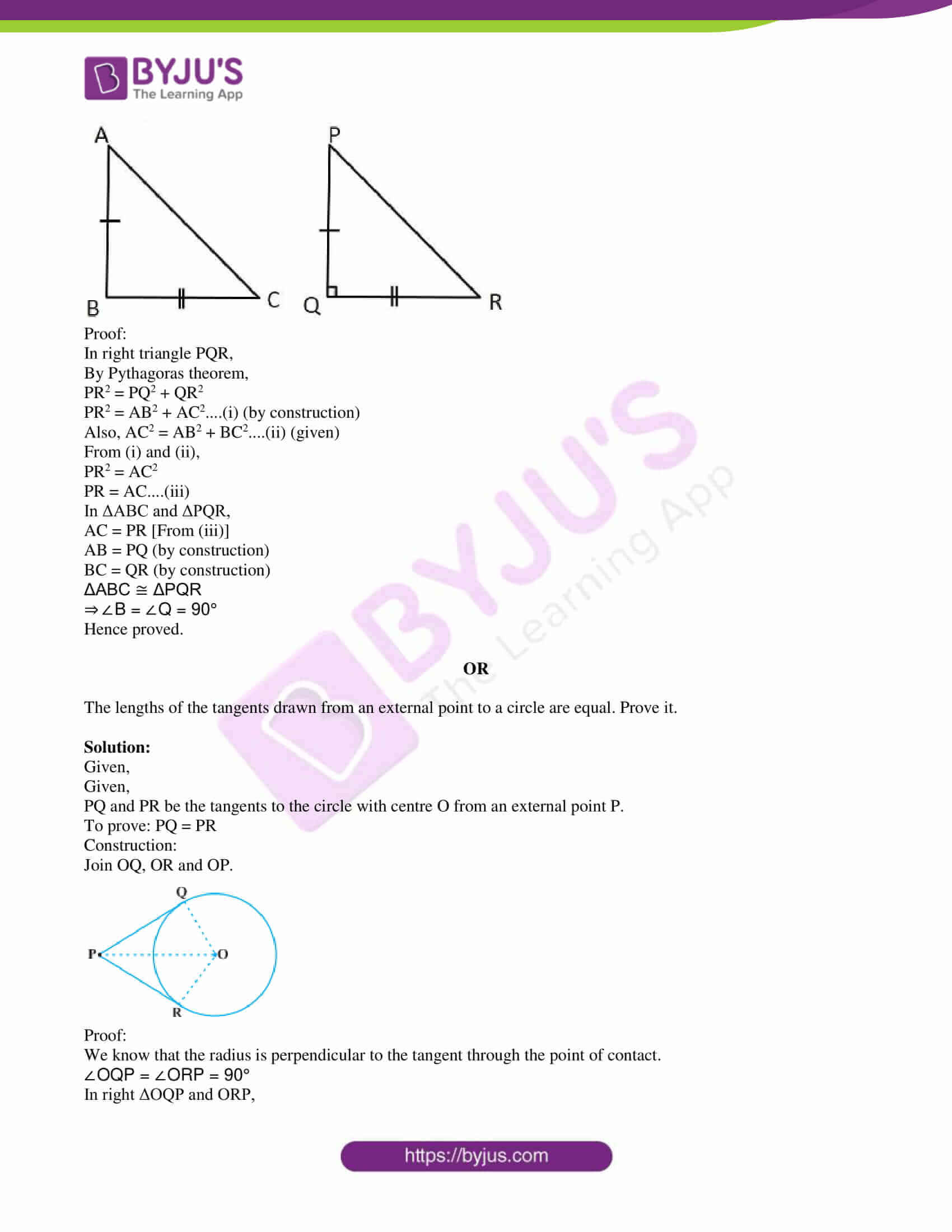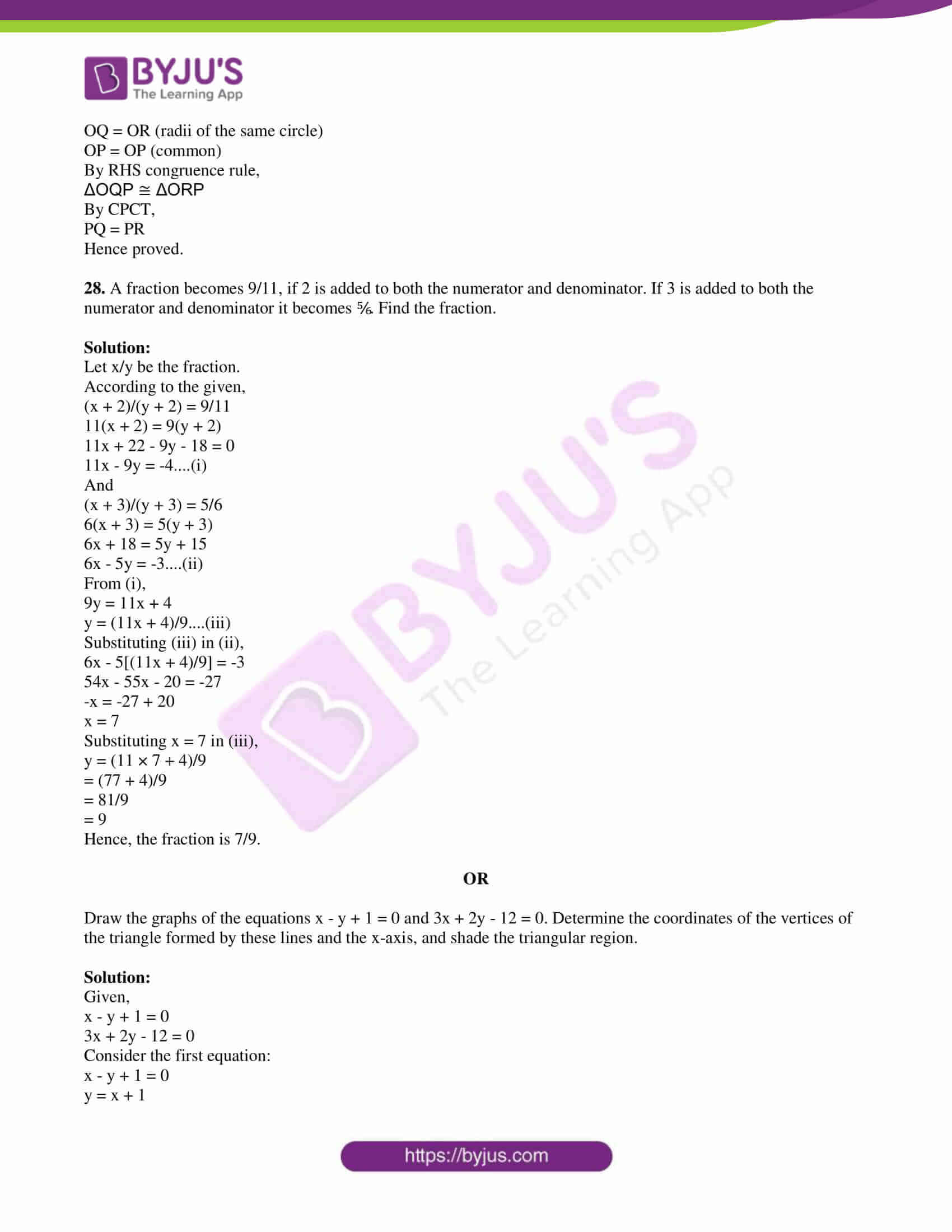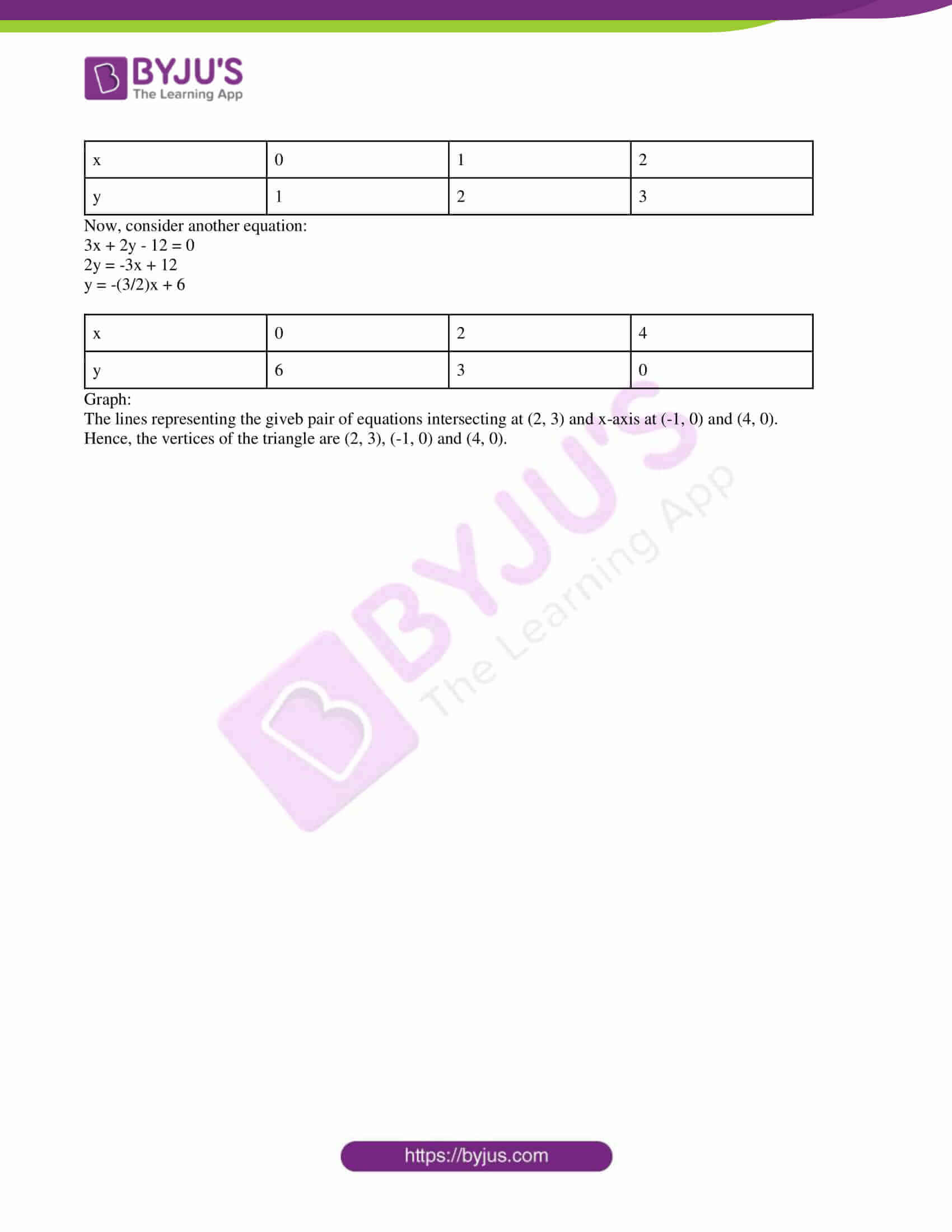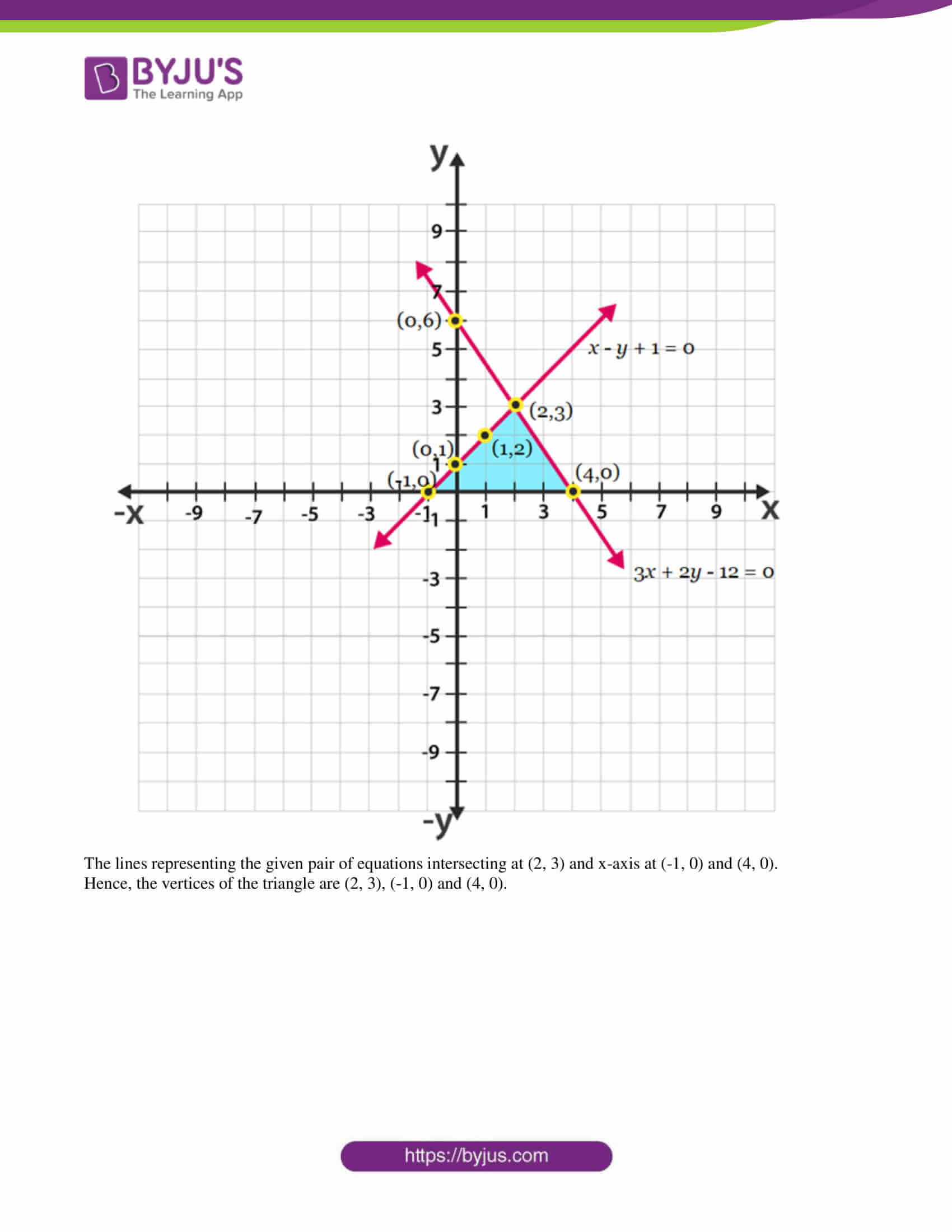Paper Code – 04/C

PART – A

1. Select the correct answer in the following:

Area of a sector of angle p (in degrees) of a circle with radius R is:

(a) (p/180) × 2πR

(b) (p/180) × πR2

(c) (p/360) × 2πR

(d) (p/720) × 2πR2

Solution:

Area of sector = (p/360) × πR2

Multiply and divide by 2,

= (p/720) ×2 πR2

2. Which of the following cannot be the probability of an event?

(a) 2/3

(b) -1.5

(c) 15%

(d) 0.7

Solution:

Probability an event cannot be negative and it can be a fraction, percentage and a whole number.

Hence, -1.5 is not possible.

3.True/False

Every composite number can be expressed (factorized) as a product of primes.

Solution:

True

Every composite number can be expressed (factorized) as a product of primes.

4. Find the first term a and common difference d of AP: -5, -1, 3, 7,…..

Solution:

Given,

-5, -1, 3, 7,…

First term = a = -5

Common difference = d = -1 – (-5) = -1 + 5 = 4

5. Write the formula for finding volume of a frustum of a cone.

Solution:

Volume of frustum of a cone = π/3 [R2 + r2 + Rr]

where, R > r (R, r = radii of circular ends)

6. Fill in the blank.

If the area of a triangle is 0 square units, then the vertices of a triangle are ______

Solution:

If the area of a triangle is 0 square units, then the vertices of a triangle are collinear.

7. Write True/False

sin (A + B) = sin A + sin B

Solution:

False

Reason: sin (A + B) = sin A cos B + cos A sin B

8. Fill in the blank.

A polynomial of degree ______ is called a linear polynomial.

Solution:

A polynomial of degree 1 is called a linear polynomial.

PART – B

9. If tangents PA and PB from a point P to a circle with centre O are inclined to each other at an angle of 80°, then find the value of ∠POA.

Solution:

Given,

∠APB = 80°

In ΔOAP and ΔOBP,

OA = OB (radii of the same circle)

PA = PB (tangents from P)

OP = OP (common)

ΔOAP ≅ ΔOBP

∠OPA = ∠OPB = 1/2 ∠APB = 1/2 (80°) = 40°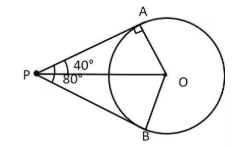In triangle OPA,

∠POA + ∠OPA + ∠OAP = 180°

∠POA + 40° + 90° = 180°

∠POA + 130° = 180°

∠POA = 180° – 130°

∠POA = 50°

10. A child has a die whose six faces show the letters as given below.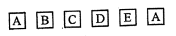The die is thrown once. What is the probability of getting

(i) A

(ii) D?

Solution:

Total number of outcomes = 6

i.e. {A, A, B, C, D, E}

(i) P(getting A) = 2/6 = ⅓

(ii) P(getting D) = ⅙

11. Use Euclid’s division algorithm to find the HCF of 420 and 130.

Solution:

420 > 130

By Euclid’s division algorithm,

420 = 130 × 3 + 30

130 = 30 × 4 + 10

30 = 10 × 3 + 0

Remainder is 0.

HCF (420, 130) = 10

12. Solve the pair of linear equations 2x + 3y = 11 and 2x – 4y = -24.

Solution:

Given,

2x + 3y = 11….(i)

2x – 4y = -24….(ii)

Subtracting (ii) from (i),

2x + 3y – (2x – 4y) = 11 – (-24)

3y + 4y = 11 + 24

7y = 35

y = 35/7

y = 5

Substituting y = 5 in (i),

2x + 3(5) = 11

2x = 11 – 15

2x = -4

x = -4/2

x = -2

Therefore, x = -2 and y = 5.

13. The wickets taken by a bowler in 10 cricket matches are as follows:

2 6 4 5 0 2 1 3 2 3

Find the mode of the data.

Solution:

Given,

2 6 4 5 0 2 1 3 2 3

2 occurs more number of times than other numbers.

Therefore, mode = 2

14. Two poles of heights 6 m and 11 m stand on a plane ground. If the distance between the feet of the poles is 12 m, find the distance between their tops.

Solution:

Let AB and CD be the two poles.

BD = Distance between the feet of the poles = 12 mIn right triangle AEC,

AC2 = AE2 + CE2

= (5)2 + (12)2

= 25 + 144

= 169

AC = 13 m

Hence, the distance between the tops of the poles is 13 m.

15. Find the discriminant of the quadratic equation 2x2 – 6x + 3 = 0 and hence find the nature of its roots.

Solution:

Given,

2x2 – 6x + 3 = 0

Comparing with the standard form ax2 + bx + c = 0,

a = 2, b = -6, c = 3

Discriminant = b2 – 4ac

= (-6)2 – 4(2)(3)

= 36 – 24

= 12 > 0

Hence, the roots of the given quadratic equation are real and distinct.

16. Divide the polynomial p(x) = x3 – 3x2 + 5x – 3 by the polynomial g(x) = x2 – 2. Find the quotient and remainder.

Solution:

Given,

p(x) = x3 – 3x2 + 5x – 3

g(x) = x2 – 2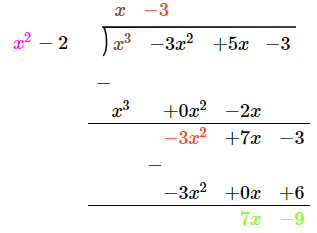Quotient = q(x) = x – 3

Remainder = r(x) = 7x – 9

PART – C

17. The angle of elevation of the top of a tower from a point on the ground which is 30 m away from the foot of the tower is 30°. Find the height of the tower.

Solution:

Let AB be the tower.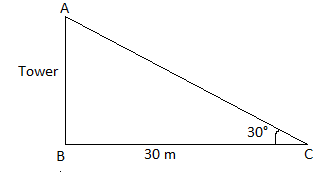In right triangle ABC,

tan 30° = AB/BC

1/√3 = AB/30

AB = 30/√3

= (30/√3) × (√3/√3)

= (30√3)/3

= 10√3

Hence, the height of the tower is 10√3 m.

18. In the given figure, OACB is a quadrant of a circle with centre O and radius 3.5 cm. If OD = 2 cm, find the area of the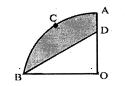Solution:

Given,

Radius = OA = OB = r = 3.5 cm

OD = 2 cm

We know that the quadrant subtends 90° at the centre O.

Area of quadrant OACB = (90°/360°) × πr2

= (1/4) × (22/7) × 3.5 × 3.5

= (1/4) × (22/7) × (7/2) × (7/2)

= 77/8

= 9.625 cm2

Area of triangle OBD,

= (1/2) × OB × OD

= (1/2) × 3.5 × 2

= 3.5 cm

Area of the shaded region = Area of quadrant OACB – Area of triangle OBD

= 9.625 – 3.5

= 6.125 cm2

19. Prove that opposite sides of a quadrilateral circumscribing a circle subtend supplementary angles at the centre of the circle.

Solution:

Given,

Quadrilateral ABCD which circumscribes a circle with centre O such that it touches the circle at point P, Q, R, and S.

To prove: ∠AOB + ∠COD = 180°

And

∠ BOC + ∠ DOA = 180°

Construction:

Join OA, OB, OC, OD, OP, OQ, OR and OS.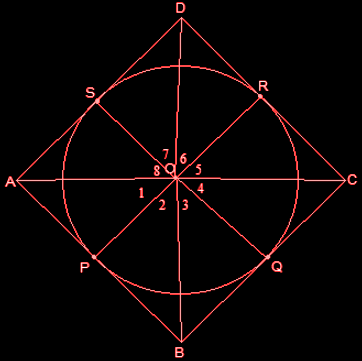Proof:

In triangles OAP and OAS,

AP = AS (tangents from the same point A)

OA = OA (common side)

OP = OS (radii of the same circle)

By SSS congruence rule,

△OAP ≅ △OAS

So, ∠POA = ∠AOS

⇒ ∠1 = ∠8

Similarly,

∠4 = ∠5

∠2 = ∠3

∠6 = ∠7

∠1 + ∠2 + ∠3 + ∠4 + ∠5 + ∠6 + ∠7 +∠8 = 360°

Now by rearranging,

⇒ (∠1 + ∠8) + (∠2 + ∠3) + (∠4 + ∠5) + (∠6 + ∠7) = 360°

⇒ 2∠1 + 2 ∠2 + 2 ∠5 + 2 ∠6 = 360°

⇒ (∠1 + ∠2) + (∠5 + ∠6) = 180°

Thus, ∠AOB + ∠COD = 180°

Similarly,

∠ BOC + ∠ DOA = 180°

Hence proved.

OR

D and E are points on the sides CA and CB respectively of a triangle ABC right angled at C. Prove that: AE2 + BD2 = AB2 + DE2

Solution:

Given,

D and E are points on the sides CA and CB respectively of a triangle ABC right angled at C.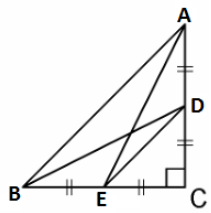In triangle ACE,

By Pythagoras theorem,

AC2 + CE2 = AE2….(i)

In triangle BCD,

BC2 + CD2 = BD2….(ii)

AC2 + CE2 + BC2 + CD2 = AE2 + BD2….(iii)

In triangle CDE,

DE2 = CD2 + CE2….(iv)

In triangle ABC,

AB2 = AC2 + CB2….(v)

Substituting (iv) and (v) in (iii),

DE2 + AB2 = AE2 + BD2

Hence proved.

20. In a class test, the sum of Shefali’s marks in Mathematics and English is 30. Had she got 2 marks more in Mathematics and 3 marks less in English, the product of their marks would have been 210. Find her marks in the two subjects.

Solution:

Let x be the marks scored in Mathematics and y be the marks scored in English.

According to the given,

x + y = 30

y = 30 – x….(i)

(x + 2)(y – 3) = 210

(x + 2)(30 – x – 3) = 210

(x + 2)(27 – x) = 210

27x – x2 + 54 – 2x = 210

x2 – 25x – 54 + 210 = 0

x2 – 25x + 156 = 0

x2 – 13x – 12x + 156 = 0

x(x – 13) – 12(x – 13) = 0

(x – 12)(x – 13) = 0

x = 12, x = 13

Substituting the value of x in (i),

When x = 12,

y = 30 – 12 = 18

When x = 13,

y = 30 – 13 = 17

Hence, the marks of Shefali in Mathematics and English are 12 and 18 or 13 and 17 respectively.

21. Consider ΔACB, right angled at C, in which AB = 29 units, BC = 21 units and ∠ABC = θ (see figure). Determine the value of sin2θ + cos2θ.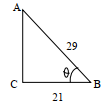Solution:

Given,

AB = 29 units

BC = 21 units

By Pythagoras theorem,

AB2 = AC2 + BC2

AC2 = (29)2 – (21)2

= 841 – 441

= 400

AC = 20 units

sin θ = AC/AB = 20/29

cos θ = BC/AB = 21/29

sin2θ + cos2θ = (20/29)2 + (21/29)2

= (400/841) + (441/841)

= (400 + 441)/841

= 841/841

= 1

Therefore, sin2θ + cos2θ = 1

OR

Prove that (1 + sec A)/sec A = sin2A/ (1 – cos A), angle A is an acute angle.

Solution:

LHS = (1 + sec A)/sec A

= [1 + (1/cos A)]/ (1/cos A)

= [(cos A + 1)/ cos A] × cos A

= 1 + cos A

= (1 + cos A) × [(1 – cos A)/ (1 – cos A)]

= (1 – cos2A)/ (1 – cos A)

= sin2A/(1 – cos A)

= RHS

Hence proved.

22. An AP consists of 50 terms of which 3rd term is 12 and the last term is 106. Find the 29th term.

Solution:

Given,

n = 50

Third term = a3 = 12

Last term = a50 = 106

nth term of AP

an = a + (n – 1)d

a3 = a + 2d

a + 2d = 12….(i)

a50 = a + 49d

a + 49d = 106….(ii)

Subtracting (i) from (ii),

49d – 2d = 106 – 12

47d = 94

d = 94/47

d = 2

Substituting d = 2 in (i)

a + 2(2) = 12

a = 12 – 4 = 8

a29 = a + 28d

= 8 + 28(2)

= 8 + 56

= 64

Therefore, 29th term of the AP is 64.

23. If A and B are (-2, -2) and (2, -4) respectively, find the coordinates of P such that AP = 3/7 AB and P lies on the line segment AB.

Solution:

Given,

AP = 3/7 AB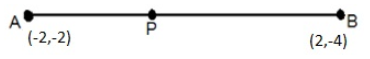Therefore, AP : PB = 3 : 4

That means, P divides AB in the ratio 3 : 4.

Here,

(x1, y1) = (-2, -2)

(x2, y2) = (2, -4)

m : n = 3 : 4

Using section formula,

P(x, y) = [(mx2 + nx1)/ (m + n), (my2 + ny1)/ (m + n)]

= [(6 – 8)/(3 + 4), (-12 – 8)/(3 + 4)]

= (-2/7, -20/7)

Hence, the coordinates of P = (-2/7, -20/7)

24. Draw a circle of radius 6 cm. From a point 10 cm away from its centre, construct the pair of tangents to the circle and measure their lengths.

Solution: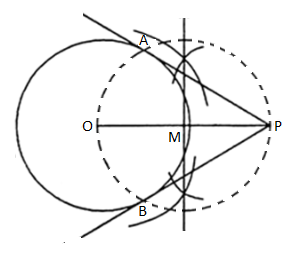Hence, PA and PB are the required tangents to the circle.

Length of the tangents = PA = PB = 8 cm

PART – D

25. The given distribution shows the number of runs scored by some top batsmen of the world in one-day international cricket matches.

 Runs scored Number of batsmen 3000 – 4000 4 4000 – 5000 18 5000 – 6000 9 6000 – 7000 7 7000 – 8000 6 8000 – 9000 3 9000 – 10000 1 10000 – 11000 1

Find the mode of the data.

Solution:

From the given data,

Maximum frequency is 18 which lies in the class interval 4000 – 5000.

Modal class = 4000 – 5000

Lower limit of the modal class = l = 4000

Frequency of the modal class = f1 = 18

Frequency of the class preceding the modal class = f0 = 4

Frequency of the class succeeding the modal class = f2 = 9

Class height = h = 1000

Mode = l + [(f1 – f0)/ (2f1 – f0 – f2)] × h

= 4000 + [(18 – 4)/ (2 × 18 – 4 – 9)] × 1000

= 4000 + [14/ (36 – 13)] × 1000

= 4000 + (14/23) × 1000

= 4000 + 608.69

= 4608.69

OR

The following distribution gives the daily income of 50 workers of a factory.

 Daily income (in Rs.) 100 – 120 120 – 140 140 – 160 160 – 180 180 – 200 No. of workers 12 14 8 6 10

Convert the distribution above to a less than type cumulative frequency distribution and draw its ogive.

Solution:

Less than type cumulative frequency distribution table:

 Daily income (in Rs.) Number of workers Less than 120 12 Less than 140 26 Less than 160 34 Less than 180 40 Less than 200 50

Plot the points (120, 12), (140, 26), (160, 34), (180, 40) and (200, 50).

Ogive: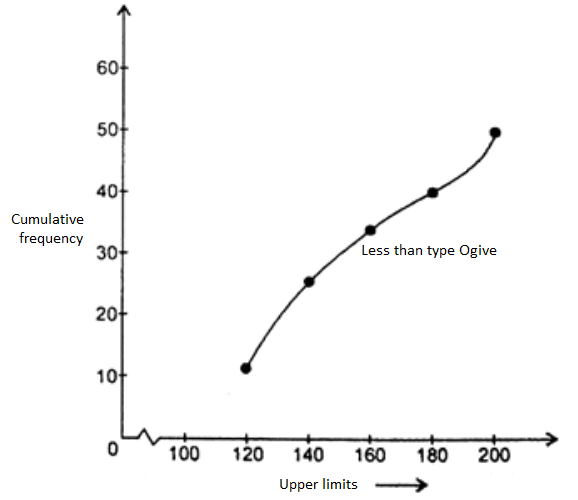26. A well of diameter 3 m is dug 14 m deep. The earth taken out of it has been spread evenly all around in the shape of a circular ring of width 4 m to form an embankment. Find the height of the embankment.

Solution:

Given,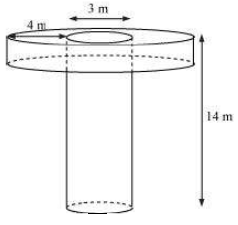Depth of the cylindrical well = h1 = 14 m

Radius of the well = r1 = 3/2 = 1.5 m

Width of embankment = 20 m

Inner radius of the embankment = r = 3/2 = 1.5 m

Outer radius of the embankment = R = Inner radius + Width

= 1.5 + 5

= 5.5 m

Let h be the height of embankment.

Volume of the cylindrical well = Volume of hollow cylindrical embankment

πr12h1 = πh(R2 – r2)

r12h1 = h(R2 – r2)

h = [(1.5)2 × 14]/ [(5.5)2 – (1.5)2]

= (2.25 × 14)/ (30.25 – 2.25)

= 1.125

Hence, the height of the embankment is 1.125 m.

OR

A juice seller was serving his customers using glasses as shown in figure. The inner diameter of the cylindrical glass was 5 cm, but the bottom of the glass had a hemispherical raised portion which reduced the capacity of the glass. If the height of the glass was 10 cm, find the apparent capacity of the glass and its actual capacity. (use π = 3.14)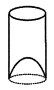Solution:

Given,

Diameter of the glass = 5 cm

Radius = r = 5/2 = 2.5 cm

Radius of glass = Radius of hemisphere = r = 2.5 cm

Height of the glass = h = 10 cm

Apparent capacity of the glass = Volume of cylinder

Actual capacity of glass = Volume of cylinder – Volume of hemisphere

= πr2h – (2/3)πr3

= π[2.5 × 2.5 × 10 – (2/3) × 2.5 × 2.5 × 2.5]

= 3.14 × [62.5 – (2/3) × 15.62]

= 196.25 – 32.7

= 163.54

Hence, the apparent capacity of the glass is 196.25 cm3 and the actual capacity is 163.54 cm3.

27. In a triangle, if square if one side is equal to the sum of the squares of the other two sides, then the angle opposite the first side is a right angle. Prove it.

Solution:

Given,

In triangle ABC,

AC2 = AB2 + BC2

To prove: ∠B = 90°

Construction:

Draw a triangle PQR right angled at Q such that PQ = AB and QR = BC.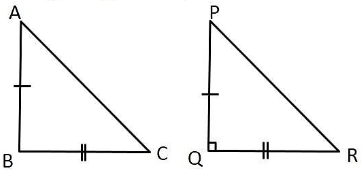Proof:

In right triangle PQR,

By Pythagoras theorem,

PR2 = PQ2 + QR2

PR2 = AB2 + AC2….(i) (by construction)

Also, AC2 = AB2 + BC2….(ii) (given)

From (i) and (ii),

PR2 = AC2

PR = AC….(iii)

In ΔABC and ΔPQR,

AC = PR [From (iii)]

AB = PQ (by construction)

BC = QR (by construction)

ΔABC ≅ ΔPQR

⇒ ∠B = ∠Q = 90°

Hence proved.

OR

The lengths of the tangents drawn from an external point to a circle are equal. Prove it.

Solution:

Given,

Given,

PQ and PR are the tangents to the circle with centre O from an external point P.

To prove: PQ = PR

Construction:

Join OQ, OR and OP.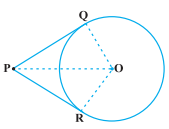Proof:

We know that the radius is perpendicular to the tangent through the point of contact.

∠OQP = ∠ORP = 90°

In right ΔOQP and ORP,

OQ = OR (radii of the same circle)

OP = OP (common)

By RHS congruence rule,

ΔOQP ≅ ΔORP

By CPCT,

PQ = PR

Hence proved.

28. A fraction becomes 9/11, if 2 is added to both the numerator and denominator. If 3 is added to both the numerator and denominator it becomes ⅚. Find the fraction.

Solution:

Let x/y be the fraction.

According to the given,

(x + 2)/(y + 2) = 9/11

11(x + 2) = 9(y + 2)

11x + 22 – 9y – 18 = 0

11x – 9y = -4….(i)

And

(x + 3)/(y + 3) = 5/6

6(x + 3) = 5(y + 3)

6x + 18 = 5y + 15

6x – 5y = -3….(ii)

From (i),

9y = 11x + 4

y = (11x + 4)/9….(iii)

Substituting (iii) in (ii),

6x – 5[(11x + 4)/9] = -3

54x – 55x – 20 = -27

-x = -27 + 20

x = 7

Substituting x = 7 in (iii),

y = (11 × 7 + 4)/9

= (77 + 4)/9

= 81/9

= 9

Hence, the fraction is 7/9.

OR

Draw the graphs of the equations x – y + 1 = 0 and 3x + 2y – 12 = 0. Determine the coordinates of the vertices of the triangle formed by these lines and the x-axis, and shade the triangular region.

Solution:

Given,

x – y + 1 = 0

3x + 2y – 12 = 0

Consider the first equation:

x – y + 1 = 0

y = x + 1

 x 0 1 2 y 1 2 3

Now, consider another equation:

3x + 2y – 12 = 0

2y = -3x + 12

y = -(3/2)x + 6

 x 0 2 4 y 6 3 0

Graph:

The lines represent the given pair of equations intersecting at (2, 3) and x-axis at (-1, 0) and (4, 0).

Hence, the vertices of the triangle are (2, 3), (-1, 0) and (4, 0).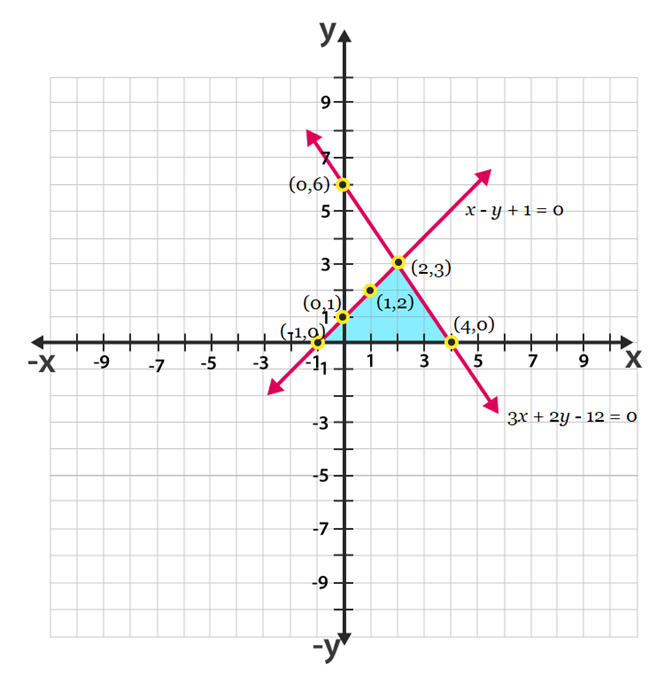The lines represent the given pair of equations intersecting at (2, 3) and x-axis at (-1, 0) and (4, 0).

Hence, the vertices of the triangle are (2, 3), (-1, 0) and (4, 0).